RS Aggarwal Class 8 Solutions Chapter 23 - Pie Charts

RS Aggarwal Class 8 Chapter 23 - Pie Charts Solutions Free PDF

RS Aggarwal class 8 solutions chapter 23 pie charts are provided here for free. A pie chart is a circular statistical graph, which is divided into slices to show numerical proportions. In the pie charts, the length of an arch in each slice is proportional to the quantity it represents. Students of class 8 CBSE are advised to solve and learn the concepts of pie charts by solving the questions from NCERT textbooks as well as from the RS Aggarwal for class 8.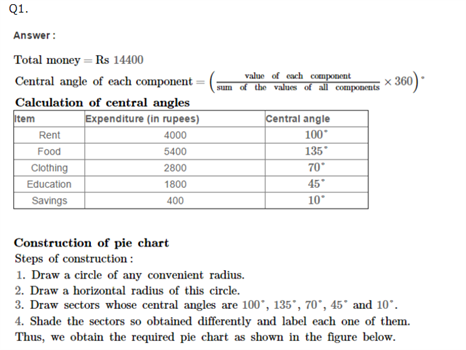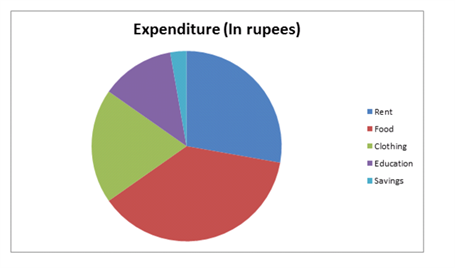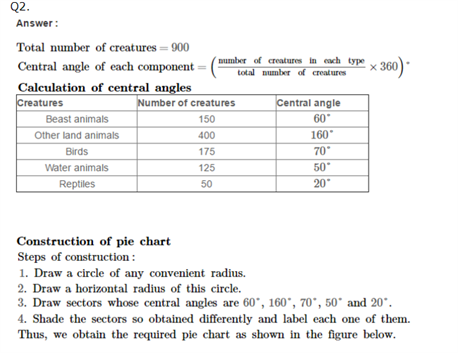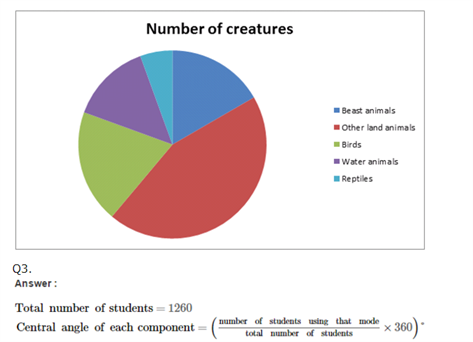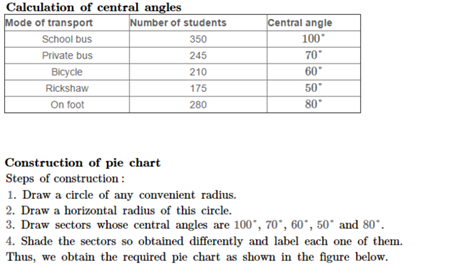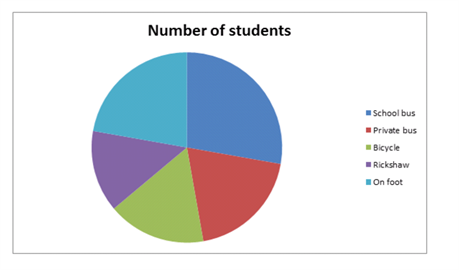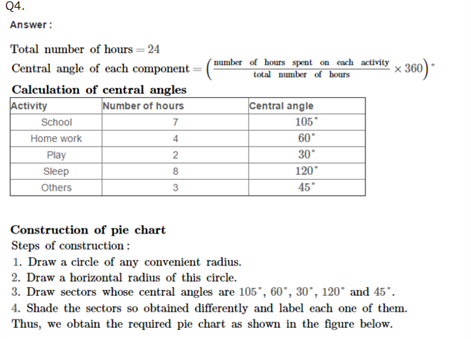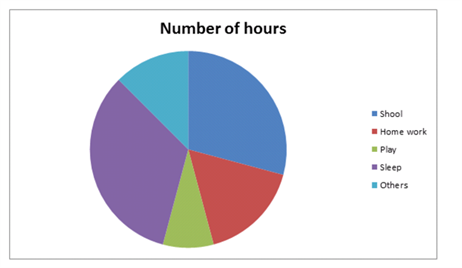Practise This Question

Being a responsible citizen, we have to develop habits that are environment friendly. What is the 4R principle we all should follow?